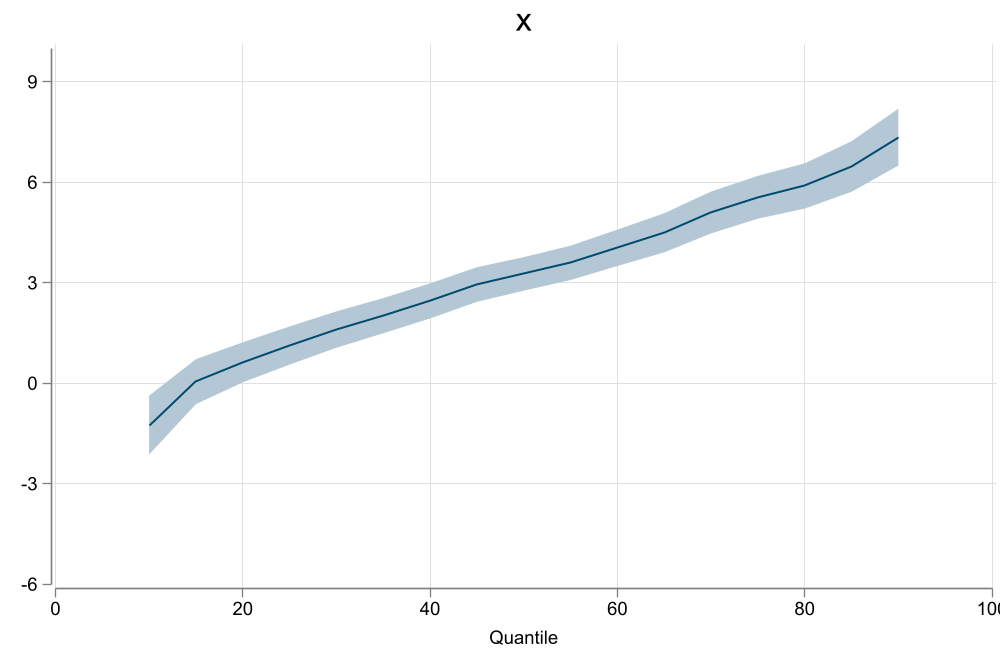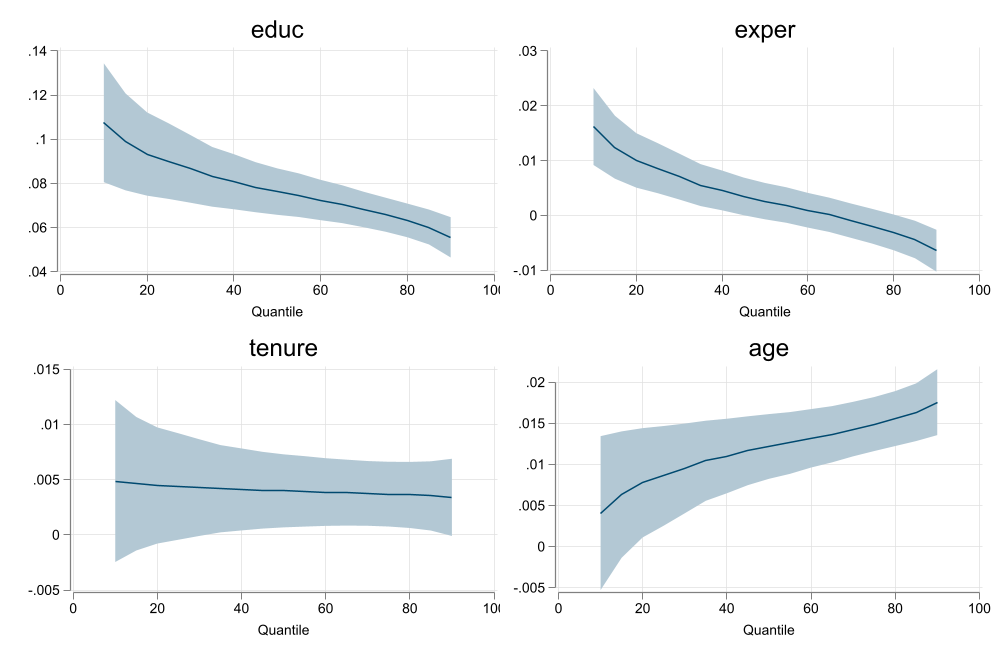# Quantile regressions with multiple fixed effects

A Method of Moments Approach

Authors

Fernando Rios-Avila

Gustavo Canavire-Bacarreza

Leonardo Siles

# Introduction

## What do Quantile Regressions do?

• Quantile Regressions are an alternative to standard linear regressions that help us to better understand the relationship between the distribution of $$Y$$ and $$X's$$.

• In constrast with Linear regression models, where one focuses on explaining $$E(y|X)$$ as a function of $$X$$, quantile regressions aim to assess the relationship between $$Q_\tau(y|X)$$ with respect to $$X$$.

• This means that to interpret Q-regressions properly, one needs to “condition” on $$X$$ but also on $$\tau$$.
• Quantile Regressions are nonlinear-models, that “look” and can be interpreted as linear ones.

Number of observations (_N) was 0, now 250.## Estimation

• A Generalization of Quantile regression data generating function can be written as follows:

$y_i = \beta_0(\tau)+\beta_1(\tau) X_{i,1}+\beta_2(\tau) X_{i,2}+...+\beta_k(\tau) X_{i,k}$

• Base on this specification, few characteristics should be considered:

1. Slopes $$\beta_k(\tau)$$ will vary across quantiles only if the model is Heteroskedastic.
2. Qreg can be considered as a semi-parametric-varying coefficient model, with unobserved running variable ($$\tau$$).
3. Coefficients are percentile specific, but functional forms can be used for more efficient estimation.
4. It is possible to separate location effects (mean) from scale effect (deviation from the mean)

Standard

$\beta(\tau) \leftarrow \frac 1 n \sum \left[ I(x_i\beta(\tau) \geq y_i) - \tau \right] =0$

Semiparamatric (Kaplan (2022))

$\beta(\tau) \leftarrow \frac 1 n \sum \left[ F\left(\frac{x_i\beta(\tau)-y_i}{bw} \right) - \tau \right] =0$

Functional (Bottai and Orsini (2019))

$\beta(\tau) = \theta_0 + \theta_1 \tau + \theta_2 \tau^2 +...$

Location-Scale $\beta(\tau) = \beta + \gamma(\tau)$# The Problem

## How to account for fixed effects?

• As said before, quantile regresions are nonlinear models. Thus, one cannot just add Dummies to address group fixed effects

$Q_\tau(y|x) = \beta_0(\tau) + \beta_1 X(\tau) + \sum \delta(\tau)_g$

This creates an incidental parameter problem. Neither $$\delta(\tau)'s$$ nor $$\beta's$$ would be consistently estimated.

• But then how to solve it?

## Some Solutions

Koenker (2004): Assume Fixed effects only have an impact on Location, and Shrink invidual effects (LASSO) $y_i = \beta(\tau)X + \delta_g$

Canay (2011): Similar to Koenker (2004), but “eliminate them” before running Qreg

\begin{aligned} y = \beta X + \delta_g + e \\ Q_\tau(y - \delta_g|X) = \beta(\tau)X \end{aligned}

Abrevaya and Dahl (2008): Correlated Random effects Model $Q_\tau(y|X) = \beta(\tau)X + \gamma(\tau) E(X|g)$

## Machado and Santos Silva (2019)

• This method was developed (and implemented using xtqreg) to incorporate individual fixed effects (Useful when considering panel data)

• In principle its an extension of He (1997), who proposed an strategy to estimate Qreg coefficients using a restricted location-scale model, assuming the following structure: $y_i = X_i\beta + \varepsilon X_i\gamma$

Thus, Quantile regression model is given by:

$Q_\tau(y|X)=X\left(\beta + F^{-1}_\varepsilon(\tau) \gamma \right) = X \beta(\tau)$

• Instead of requiring $$K\times M$$ coefficients to identify the whole conditional distribution, it only requires $$2\times K + M$$.

• Also, Quantile curves will not cross!

## How does Simplified He (1997) and Machado and Santos Silva (2019) works?

$y_i = x_i \beta + \varepsilon x_i \gamma \rightarrow Q_\tau(y|X)=X\beta + X\gamma F_\varepsilon^{-1}(\tau)$

## Extension: Multiple Fixed effects

Machado and Santos Silva (2019) propose an extension: To add a single set of fixed effect (panel) apply Frisch–Waugh–Lovell theorem.

This can be extended to controlling for multiple fixed effects:

$\tilde w_i = w_i +\bar w - \delta^w_{g1} - \delta^w_{g2} - ... - \delta^w_{gk} \ \forall \ w \in y,x$

And use almost the same moments as before:

\begin{aligned} E(\tilde X(\tilde y-\tilde X\beta)) & =0 \\ E(\tilde X(|\tilde y- \tilde X\beta|-\tilde X\gamma)) &=0 \\ X\gamma + \delta's &= y - (\tilde y- \tilde X\gamma) \\ E\left[ I\left( \frac{\tilde y- \tilde X\beta}{X\gamma+ \delta's } \geq q_\tau \right) -\tau \right] &=0 \end{aligned}

## Extension: Standard Errors

• Along with the modified algorithm that allows for Panel Fixed effects, Machado and Santos Silva (2019) proposed a GLS type variance estimator.

• The model assumes the existance of Heteroskedasticity.
• Based on Law of Iterated Expectations, and assuming Heteroskedasticity is correctly specified, its possible to construct a GLS type Variance Estimator.
• How does this work?

## Location Model

• Consider the location model: $$y_i = x_i \beta + \varepsilon_i x_i \gamma$$

• Which has known heteroskedasticity: $$Var(y_i - x_i\beta)=(x_i\gamma)^2 \sigma^2_\varepsilon$$

• Machado and Santos Silva (2019) GLS Variance would be: \begin{aligned} e &= y_i - X\beta = \varepsilon_i x_i \gamma\\ Var(\hat\beta) &= (X'X)^{-1}\sum x_i x_i' \color{red}{e_i^2} (X'X)^{-1} \\ &=\color{red}{\sigma_\varepsilon^2} (X'X)^{-1} \sum x_i x_i' \color{red}{(x_i'\gamma)^2} (X'X)^{-1} \end{aligned}

However, this can be sensitive to the modeling of the Scale function.

But one can almost always Bootstrap…

## Extension: Robust and Clustered SE

An extension offered in my implementation makes full use of the moment conditions, and the implicit Influence functions: \begin{aligned} IF'_{i,\beta} &= N(X'X)^{-1} x_i R_i \\ IF'_{i,\gamma} &= N(X'X)^{-1} x_i \Big( \tilde R_i -x_i'\gamma \Big) \\ \color{blue}{IF_{i, q(\tau)}} &= \frac{\tau -I(q_\tau \geq \varepsilon_i) }{f_\varepsilon(q_\tau)} - \frac{R_i}{\bar x'\gamma} - q_\tau \frac{\tilde R_i -x_i'\gamma}{\bar x'\gamma}\\ R_i &= y_i-x_i'\beta \\ \tilde R_i & =2 R_i \big[1(R_i \geq 0) - E(1(R_i \geq 0)) \big] \end{aligned}

So we just stack Influence functions to obtain Robust and Clustered Standard errors:

Robust SE $Var_r(\beta,\gamma,q_\tau) = \frac{1}{N^2} \left[ \begin{matrix} IF_\beta & IF_\gamma & IF_{q_\tau} \end{matrix} \right]'\left[ \begin{matrix} IF_\beta & IF_\gamma & IF_{q_\tau} \end{matrix} \right]$

Cluster SE

$Var_c(\beta,\gamma,q_\tau) = \frac{1}{N^2} \left[ \begin{matrix} SIF_\beta & SIF_\gamma & SIF_{q_\tau} \end{matrix} \right]'\left[ \begin{matrix} SIF_\beta & SIF_\gamma & SIF_{q_\tau} \end{matrix} \right]$ $SIF_{w} = \left[ \begin{matrix} SIF_{g=1,w} \\ SIF_{g=2,w} \\ ... \\\ SIF_{g=G,w} \end{matrix}\right] \& SIF_{g=k, \ w} = \sum_{i \in g=k} IF_{i,w}$

Easily allows for simultaneous Qregressions and weights

## Command syntax mmqreg

mmqreg depvar indepvar [if in] [pw] , [Options] <-- Standard Syntax

** Variance Options
Default: MSS(2019) GLS SE
-robust-: Robust Standard errors, no DOF Correction (GMM)
-cluster(varname)-: Clustered Standard errors, no DOF Correction (GMM)

** Output
- q(numlist): List of Quantiles to be estimated (default q=50)
- ls        : Request Providing Location Scale coefficient models

There will be some differences with xtqreg because of couple of differences in the math and programming.

## Example

webuse nlswork, clear
qui:mmqreg ln_w age ttl_exp tenure not_smsa south, ///
abs(idcode)
est sto m1
qui:mmqreg ln_w age ttl_exp tenure not_smsa south, ///
abs(idcode) robust
est sto m2
qui:mmqreg ln_w age ttl_exp tenure not_smsa south, ///
abs(idcode) cluster(idcode)
est sto m3
qui:mmqreg ln_w ttl_exp tenure not_smsa south, ///
abs(idcode age) cluster(idcode)
est sto m4
esttab m1 m2 m3 m4, se  nomtitle nogap 
(National Longitudinal Survey of Young Women, 14-24 years old in 1968)

----------------------------------------------------------------------------
(1)             (2)             (3)             (4)
----------------------------------------------------------------------------
qtile
age              -0.00270**      -0.00270**      -0.00270*
(0.000868)      (0.000890)       (0.00127)
ttl_exp            0.0292***       0.0292***       0.0292***       0.0341***
(0.00178)       (0.00153)       (0.00225)       (0.00229)
tenure             0.0108***       0.0108***       0.0108***       0.0102***
(0.00191)      (0.000989)       (0.00145)       (0.00144)
not_smsa          -0.0925***      -0.0925***      -0.0925***      -0.0880***
(0.00974)        (0.0104)        (0.0140)        (0.0138)
south             -0.0640***      -0.0640***      -0.0640***      -0.0599***
(0.0116)        (0.0121)        (0.0167)        (0.0167)
_cons               1.611***        1.611***        1.611***        1.490***
(0.0564)        (0.0194)        (0.0276)        (0.0146)
----------------------------------------------------------------------------
N                   28093           28093           28093           28093
----------------------------------------------------------------------------
Standard errors in parentheses
* p<0.05, ** p<0.01, *** p<0.001
sysuse auto, clear
qui:mmqreg price mpg trunk  ,
est sto m1
qui:mmqreg price mpg trunk  , robust
est sto m2
qui:bootstrap, reps(250):mmqreg price mpg trunk
est sto m3
(1978 automobile data)

------------------------------------------------------------
(1)             (2)             (3)
default          Robust       Bootstrap
------------------------------------------------------------
qtile
mpg                -172.7          -172.7***       -172.7*
(38416.1)         (44.85)         (71.63)
trunk               33.67           33.67           33.67
(50437.7)         (49.04)         (56.11)
_cons              8457.3          8457.3***       8457.3***
(1454004.1)        (1617.9)        (2303.5)
------------------------------------------------------------
N                      74              74              74
------------------------------------------------------------
Standard errors in parentheses
* p<0.05, ** p<0.01, *** p<0.001

## Plotting: qreg vs mmqreg## Conclusions

• In this presentation I present a quick review of quantile regressions, with emphasis on solutions for adding fixed effects.

• The Location and Scale model help with the problem because it reduces the number of coefficients to needed to be estimated for consistent estimates.

• In MSS2019, inconsistencies are still observed with Small T. (get long data)
• In my context, this may be less biding, because is more likely to have many obs per Fixed characteristic.
• While the model may be restrictive (multiplicative heteroskedasticity) it may still be useful for exploration, and analysis when other methodologies are not feasible.